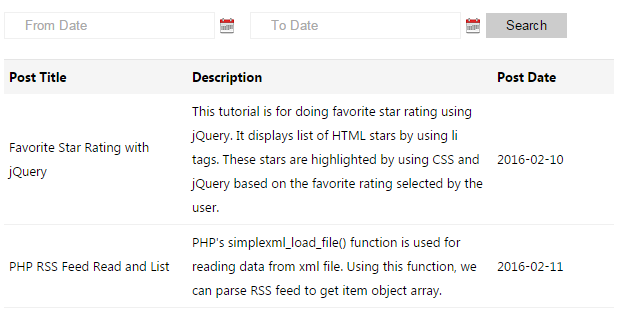# PHP MySQL Date Range Search with jQuery DatePicker

In this tutorial, we are going to see how to search database records date between two given ranges. It will return the filtered results from the database based on these dates input.

In this tutorial, we are using jQuery DatePicker to choose the dates for the search options. These date inputs are used to form a database query to read rows within two dates by the use of BETWEEN clause.

View Demo

## HTML Date Range Search Form

This code shows the HTML code for displaying database records and the search input controls. These controls are having a DatePicker to select date input.``````<form name="frmSearch" method="post" action="">
<p class="search_input">
<input type="text" placeholder="From Date" id="post_at" name="search[post_at]"  value="<?php echo \$post_at; ?>" class="input-control" />
<input type="text" placeholder="To Date" id="post_at_to_date" name="search[post_at_to_date]" style="margin-left:10px"  value="<?php echo \$post_at_to_date; ?>" class="input-control"  />
<input type="submit" name="go" value="Search" >
</p>
</form>
``````

## PHP MySQL Date Range Search Filter

This code reads dates from the user for the search form controls and creates a database query to filter records based on the date fields.

``````<?php
\$conn = mysqli_connect("localhost", "root", "", "blog_samples");

\$post_at = "";
\$post_at_to_date = "";

\$queryCondition = "";
if(!empty(\$_POST["search"]["post_at"])) {
\$post_at = \$_POST["search"]["post_at"];
list(\$fid,\$fim,\$fiy) = explode("-",\$post_at);

\$post_at_todate = date('Y-m-d');
if(!empty(\$_POST["search"]["post_at_to_date"])) {
\$post_at_to_date = \$_POST["search"]["post_at_to_date"];
list(\$tid,\$tim,\$tiy) = explode("-",\$_POST["search"]["post_at_to_date"]);
\$post_at_todate = "\$tiy-\$tim-\$tid";
}

\$queryCondition .= "WHERE post_at BETWEEN '\$fiy-\$fim-\$fid' AND '" . \$post_at_todate . "'";
}

\$sql = "SELECT * from posts " . \$queryCondition . " ORDER BY post_at desc";
\$result = mysqli_query(\$conn,\$sql);
?>
``````